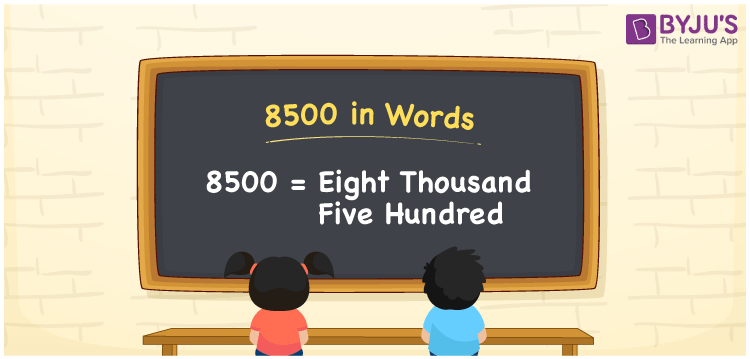# 8500 in Words

We can write 8500 in words as Eight thousand five hundred. If you won a prize worth Rs. 8500 in a game, you can say, “I won a prize in the game that worth Eight thousand five hundred rupees”. We know that 8500 is a cardinal number as it denotes a specific quantity.

 8500 in words Eight thousand five hundred Eight thousand five hundred in Numbers 8500

## 8500 in English Words

We generally write numbers in words using the English alphabet. Thus, we can read 8500 in English as “Eight thousand five hundred”.## How to Write 8500 in Words?

We can see that the number 8500 has 4 digits, so we need to make a place value chart with columns as shown below.

 Thousands Hundreds Tens Ones 8 5 0 0

Here, ones = 0, tens = 0, hundreds = 5, and thousands = 8.

Thus, we can write the expanded form as:

8 × Thousand + 5 × Hundred + 0 × Ten + 0 × One

= 8 × 1000 + 5 × 100 + 0 × 10 + 0 × 1

= 8000 + 500

= 8500

= Eight thousand five hundred

Hence, 8500 in words is written as Eight thousand five hundred.

8500 is a natural number that precedes 8501 and succeeds 8499.

8500 in words – Eight thousand five hundred

Is 8500 an odd number? – No

Is 8500 an even number? – Yes

Is 8500 a prime number? – No

Is 8500 a composite number? – Yes

Is 8500 a perfect square number? – No

Is 8500 a perfect cube number? – No

## Frequently Asked Questions on 8500 in Words

Q1

### How do you write 8500 in words?

We can write 8500 in words as Eight thousand five hundred.
Q2

### What is the spelling of 8500 in English?

The spelling of 8500 in English is “Eight thousand five hundred rupees”.
Q3

### How to write 8500 in words on a cheque?

On a cheque, we generally write 8500 in words as Eight thousand five hundred rupees only.